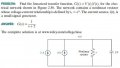# RL differential equations

#### Saitron

Joined Mar 20, 2020
13
Hello i am new on the forum and i want to ask my 1st question.I have to find the transfer function v(t)/i(t). First of all i calculate the the differential equations and the result is 2+i(t)=ir+c*dv/dt
2+i(t)=e^vr+c*dv/dt
Then i have to lenearize the e^v and the result is 2*v+1.38.
I think i have a mistake on the general idea and i can't proof that v(s)/i(s)=1/(s+2)
I will appreciate ifanyone can check it and help my to find the solution.

#### Papabravo

Joined Feb 24, 2006
18,417
Why do you think that 2*v+1.38 is the correct linear approximation to e^(v_r). Shouldn't it look like the Taylor series about the point v_r=0
It might help if you could show the intermediate steps

#### Saitron

Joined Mar 20, 2020
13
Sorry i made a typographical error 2*v+0.61 . I use the Taylor series method to lenearize it and i solve i=e^V and and i use i=2, 2=e^v so v=ln2=0.69. I hold this number V=0.69 and then i do the taylor series about the point v=0.69.

#### Papabravo

Joined Feb 24, 2006
18,417
So do you now get the correct result?
If not could please show the complete set of steps to get from the given information to your erroneous solution. Why are you resisting this request?

#### Papabravo

Joined Feb 24, 2006
18,417
Sorry, the picture is so lacking in contrast that I can't make out the parts in light blue pen. The text is too small.

#### Papabravo

Joined Feb 24, 2006
18,417
The proper approximation of an exponential function about a point a=ln(2) should be:

$f(x)\;=\;f(a)+f'(a)(x-a)+...$
$f(x)\;=\;e^{ln2}+e^{ln2}(x-ln2)\;=\;2(1-ln2+x)$

Does that help?

•Saitron

#### MrAl

Joined Jun 17, 2014
8,988
Hello,

It looks to me like you guys are making this way too hard. It's much, much simpler than that.
Do you really think you need a Taylor series to solve this when the answer is given and is so so simple?

First establish the DC operating point That provides you with the "linearization" of the non linear resistor.
Next, apply the current source assuming small signal.

However, in the "Answer" given it looks like they left off the initial conditions so the given answer is not yet complete really unless you just want to figure the small signal response which is entirely possible then it is correct.

Since the input wave is defined too generally as i(t), you would have to convolve the input with the transfer function to get the entire response. Unless that is given the best you can do is call it I(s).

#### Saitron

Joined Mar 20, 2020
13
The purpose of the exercise is to make the transfer function from the small signal i(t) and the v(t). Is the operation point of the non linear resistor the 2ampere? Because the only way to find the Vr and lenearize the the e^V is to solve 2=e^v.

#### MrAl

Joined Jun 17, 2014
8,988
The purpose of the exercise is to make the transfer function from the small signal i(t) and the v(t). Is the operation point of the non linear resistor the 2ampere? Because the only way to find the Vr and lenearize the the e^V is to solve 2=e^v.
Yes that is correct, and that's what makes it so easy to solve.
i(t) would be considered small signal which then is used to find the output response small signal.

So did you get the same answer as they did yet?
I get a slightly different result than the answer given because of the i=e^v response of the resistor. At the DC operating point the resistance is not 1 therefore RC can not equal 1 and it is not 2 and it is not 1/2 either.

Last edited:

#### Saitron

Joined Mar 20, 2020
13
I used both of the two current sources to solve the equation. i tried to solve it with only the small signal but the result wasn't the same. Why we use only the small signal to solve the equation?

#### MrAl

Joined Jun 17, 2014
8,988
I used both of the two current sources to solve the equation. i tried to solve it with only the small signal but the result wasn't the same. Why we use only the small signal to solve the equation?
You can assume small signal because they say to linearize the resistor. What that implies is that the current can not change too much through that resistor or else the initial DC bias point is no longer valid and so for a sign wave there would be distortion caused by the now changing resistance.

Yes you use both current sources, but first you use just the 2 amp one. The 2 amp source sets the resistor value. Then when i(t) comes into play later, the resistor acts like a linear resistor for small current changes.

If you wanted to do large signal, you would have to include the source i(t) into the solution but you cant do that because you dont know what it is yet, so you would never know how the resistance changes with large signal. Since this is in the frequency domain anyway, you could include i(t) in the solution by just calling it I(s) which i think you know.